﻿ 河南省极端气温时空变化特征分析 Temporal and Spatial Variation Characteristics of Extreme Temperature in Henan Province

Open Journal of Nature Science
Vol. 07  No. 06 ( 2019 ), Article ID: 32920 , 12 pages
10.12677/OJNS.2019.76064

Temporal and Spatial Variation Characteristics of Extreme Temperature in Henan Province

Lingqing Wang1,2

1School of Atmospheric Sciences, Chengdu University of Information Technology, Chengdu Sichuan

2Dingxin Meteorological Station of Jinta County, Jiuquan Gansu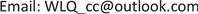Received: Oct. 21st, 2019; accepted: Nov. 5th, 2019; published: Nov. 12th, 2019ABSTRACT

The daily average data of the highest and lowest temperatures observed at 15 ground stations in Henan Province from 1958 to 2012 are used to analyze the temporal and spatial changes and abrupt changes of the extreme temperature in Henan Province more comprehensively and accurately by means of linear regression, inverse distance weighted difference and Mann Kendall (m-k) method. After analyzing, the conclusions are as follows: The extreme high temperature indices in Henan Province showed an upward trend as a whole. The change tendency rates of heat persistence index, summer days, the percentile value of warm day and the percentile value of warm night were −0.3 d/10a, 1.3 d/10a, −0.16 d/10a and 2.9 d/10a, respectively. The extremely low temperature indices showed a significant downward trend, while the cold persistence index, frost days, the percentile value of cold day and the percentile value of cold night showed a significant downward trend. The change tendency rates of the number were −0.15 d/10a, −3.4 d/10a, −0.4 d/10a, −2.4 d/10a, respectively. And the trend of climate warming in Henan Province is more obvious after 2000. The change of extreme temperature in Henan Province is asymmetric. Compared with the increase of extremely high temperature indices, the extremely low temperature indices have a larger decline. Especially compared with the rising trend of summer days, the downward trend of frost days is very significant. In the spatial variation, the range and value (absolute value) of the percentile value of warm night, frost days and the percentile value of cold night change propensity rates are significantly larger than other extreme exponents. The spatial change has the most obvious influence on the three extreme temperature indices, while the altitude changes to summer days and frost days. The impact is most pronounced. The mutation in the extreme temperature index occurred mainly around 2000.

Keywords:Extreme Temperature, Temporal and Spatial Variation Characteristics, Henan Province

1成都信息工程大学大气科学学院，四川 成都

2金塔县鼎新气象站，甘肃 酒泉1. 引言

2. 数据与方法

2.1. 数据来源与质量控制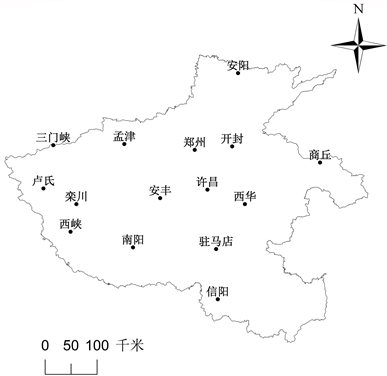Figure 1. Selection of meteorological station distribution in Henan

2.2. 研究方法Table 1. Definition of extreme temperature indices

${y}_{i}=a+b{x}_{i}\text{\hspace{0.17em}}\text{\hspace{0.17em}}\left(i=1,2,3,\cdots ,n\right)$ (1)

$Z=\underset{i=1}{\overset{n}{\sum }}\left({Z}_{i}\cdot {W}_{i}\right)/\underset{i=1}{\overset{n}{\sum }}{W}_{i}$ (2)

Mann-Kendall (M-K)突变检验对均值突变和转折突变比较敏感，具有人为干扰性少、校验范围宽的优点，因此十分适合用来检验极端气温指数的突变。首先设原始时间序列为 ${t}_{1},{t}_{2},\cdots ,{t}_{n}$${r}_{i}$ 表示第i个样本 ${t}_{i}$ 大于 ${t}_{i}$ ( $1\le j\le i$ )的累积数，则统计量计算公式为：

${d}_{k}=\underset{i=1}{\overset{k}{\sum }}{r}_{i}\left(2\le k\le n\right)$ (3)

k为样本数量； ${d}_{k}$ 为样本累积数之和。假设原序列随机独立， ${d}_{k}$ 的均值 $E\left({d}_{k}\right)$${d}_{k}$ 的方差 $\text{Var}\left({d}_{k}\right)$ 计算公式分别为：

$E\left({d}_{k}\right)=k\left(k-1\right)/4$ (4)

$\text{Var}\left({d}_{k}\right)=k\left(k-1\right)\left(2k+5\right)/12\text{\hspace{0.17em}}\text{\hspace{0.17em}}\text{\hspace{0.17em}}\left(2\le k\le n\right)$ (5)

$U{F}_{k}=\left({d}_{k}-E\left({d}_{k}\right)\right)/\sqrt{\text{Var}\left({d}_{k}\right)}\text{\hspace{0.17em}}\left(k=1,\cdots ,n\right)$ (6)

3. 结果与分析

3.1. 河南省极端气温指数时间变化特征分析Figure 2. Trend of extreme high temperature indices in Henan from 1958 to 2012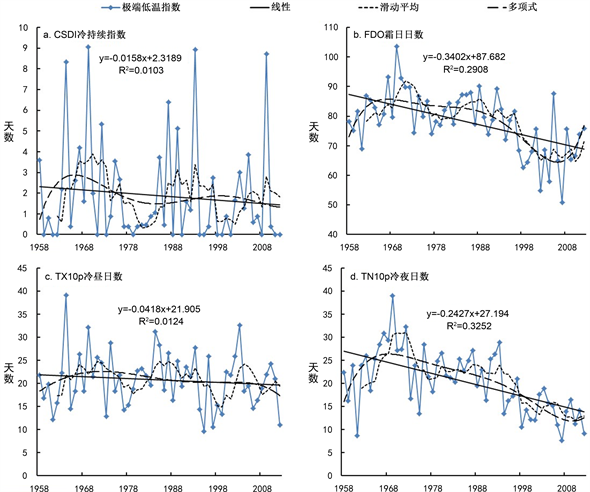Figure 3. Trend of extreme low temperature indices in Henan from 1958 to 2012

3.2. 河南省极端气温指数时间序列突变分析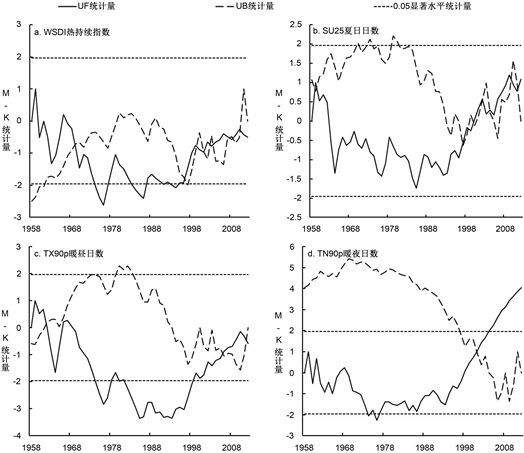Figure 4. Mann-Kendall test of extreme high temperature indices in Henan from 1958 to 2012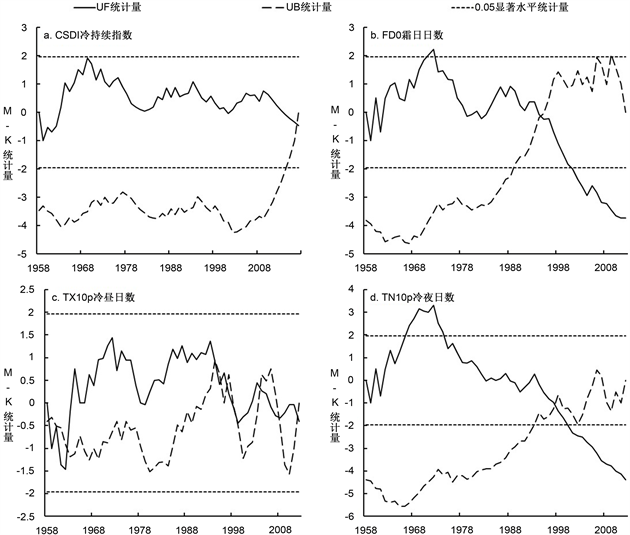Figure 5. Mann-Kendall test of extreme low temperature indices in Henan from 1958 to 2012

3.3. 河南省极端气温指数空间变化特征分析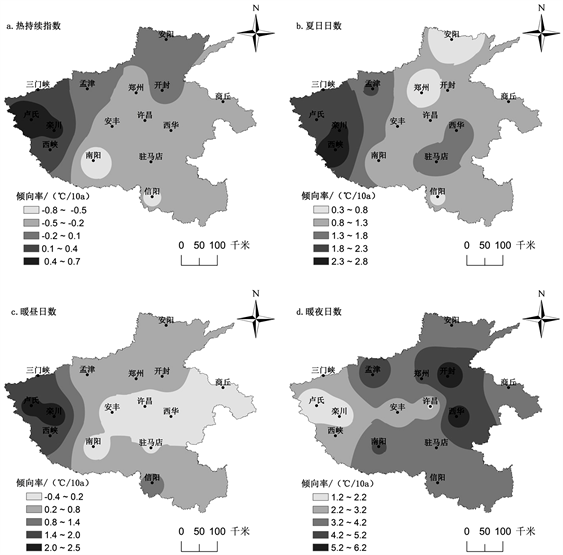Figure 6. Spatial distribution of inter-annual variation of extreme high temperature indices in Henan from 1958 to 2012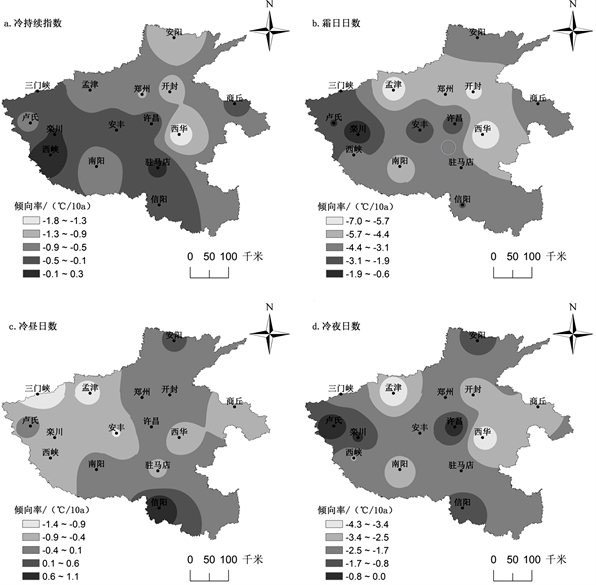Figure 7. Spatial distribution of inter-annual variation of extreme low temperature indices in Henan from 1958 to 2012Table 2. Mean trends of extreme temperature in categorized latitude ranks in Henan from 1958 to 2012

4. 结论与讨论

4.1. 结论

1) 1958~2012年河南省极端高温指数整体呈现上升趋势，极端低温指数整体呈显著下降趋势，但两者之间的变化是不对称的，与极端高温指数的上升幅度相比，极端低温指数的下降幅度更大。总体上，河南省气候变暖趋势十分显著，与全国乃至全球变暖的大背景相符。

2) 在1958~2012年之间，热持续指数、暖昼日数、冷持续指数和冷昼日数没有发现明显的突变，夏日日数通过验证认为其在1997年有一突变点，而暖夜日数、霜日日数和冷夜日数的突变点分别为2001年、1994年和1998年。综上所述，河南省极端气温指数的突变主要发生在2000年前后。

3) 在不同的地区，极端气温指数之间变化倾向率有明显的差异，暖夜日数、霜日日数和冷夜日数变化倾向率的范围和数值(绝对值)明显大于其他极端指数，相对于其他极端气温指数，区域变化对这三个极端气温指数的影响更大。

4) 在此次研究选取的8个极端气温指数中，河南省夏日日数和霜日日数受海拔的影响较明显，夏日日数随着海拔的升高而逐渐减少，霜日日数随着海拔的升高而逐渐增加。

4.2. 讨论

Temporal and Spatial Variation Characteristics of Extreme Temperature in Henan Province[J]. 自然科学, 2019, 07(06): 549-560. https://doi.org/10.12677/OJNS.2019.76064

1. 1. 任国玉. 地表气温变化研究的现状和问题[J]. 气象, 2003, 29(8): 3-6.

2. 2. 王绍武. 近百年我国及全球气温变化趋势[J]. 气象, 1990, 16(2): 11-15.

3. 3. 王琼, 张明军, 王圣杰, 等. 1962-2011 年长江流域极端气温事件分析[J]. 地理学报, 2013, 68(5): 611-625.

4. 4. Rusticucc, I.M. (2012) Observed and Simulated Variability of Extreme Temperature Events over South America. Atmospheric Research, 106, 1-17. https://doi.org/10.1016/j.atmosres.2011.11.001

5. 5. 翟盘茂, 潘晓华. 中国北方近50年温度和降水极端事件变化[J], 地理学报, 2003, 58(S1): 1-10.

6. 6. 任福民, 翟盘茂. 1951-1990年中国极端气温变化分析[J]. 大气科学, 1998, 22(2): 217-227.

7. 7. 翟盘茂, 任福民. 中国近四十年最高最低温度变化[J]. 气象学报, 1997, 55(4): 418-429.

8. 8. 尹义星, 王小军, 叶正伟, 焦士兴, 潘欣. 1951-2013年江苏省极端最高和最低气温变化趋势及概率特征[J]. 长江流域资源与环境, 2018, 27(6): 51-59.

9. 9. 任国玉, 初子莹, 周雅清, 等. 中国气温变化研究最新进展[J]. 气候与环境研究, 2005, 10(4): 701-716.

10. 10. 郭瑜. 河南省近49年来降水和气温变化特征研究[D]: [硕士学位论文]. 郑州: 郑州大学, 2012: 32-36.

11. 11. 高文华, 李开封, 崔豫. 1960-2014年河南极端气温事件时空演变分析[J]. 地理科学, 2017, 37(8): 1259-1269.

12. 12. Gongd, H. (2004) Extreme Climate Events in Northern China over the Last 50 Years. Acta Geographica Sinica, 59, 230-238.

13. 13. 朱大运, 熊康宁, 肖华. 贵州省极端气温时空变化特征分析[J]. 资源科学, 2018, 40(8): 180-191.

14. 14. 张雷, 任国玉, 刘江, 等. 城市化对北京气象站极端气温指数趋势变化的影响[J]. 地球物理学报, 2011, 54(5): 1150-1159.

15. 15. Qian, W.H. and Lin, X. (2004) Regional Trends in Recent Temperature Indices in China. Climate Research, 27, 119-134. https://doi.org/10.3354/cr027119

16. 16. 周雅清, 任国玉. 城市化对华北地区极端气温事件频率的影响[J]. 高原气象, 2014, 33(6): 1589-1598.